Profilers > Simulator > Additional Examples of the Simulator > Example of Simulating General Formulas
Publication date: 08/13/2020

## Example of Simulating General Formulas

Though the profiler and simulator are designed to work from formulas stored from a model fit, they work for any formula that is stored in a column. A typical application of simulation is to exercise financial models under certain probability scenarios to obtain the distribution of the objectives. This can be done in JMP. The key is to store the formulas into columns, set up ranges, and then conduct the simulation.

1. In the JMP Home Window, select File > New > Script. This opens a new script window.

2. Copy and paste the following JSL script into the new script window.

`dt = New Table( "Sales Model" );`
`dt << New Column( "Unit Sales", Values( {1000, 2000} ) );`
`dt << New Column( "Unit Price", Values( {2, 4} ) );`
`dt << New Column( "Unit Cost", Values( {2, 2.5} ) );`
`dt << New Column( "Revenue",`
`	Formula( :Unit Sales * :Unit Price )`
`);`
`dt << New Column( "Total Cost",`
`	Formula( :Unit Sales * :Unit Cost + 1200 )`
`);`
`dt << New Column( "Profit",`
`	Formula( :Revenue - :Total Cost ),`
`	Set Property( "Spec Limits", {LSL( 0 )} )`
`);`
`Profiler(`
`	Y( :Revenue, :Total Cost, :Profit ),`
`	Objective Formula( Profit )`
`);`

3. Click the Run Script icon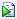to run the script. Alternatively, you can select Ctrl-R.

The script creates the data table in Figure 8.24 with some initial scaling data and stores formulas into the output variables. It also launches the Prediction Profiler.

Figure 8.24 Data Table Created from Script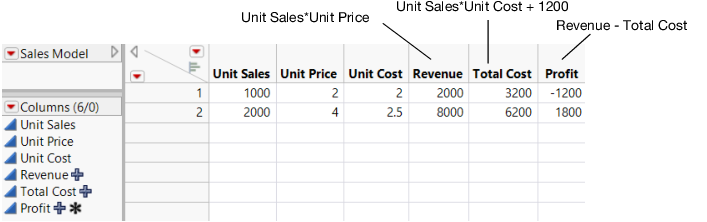4. Click the Prediction Profiler red triangle and select the Simulator.

5. In the menus beneath Unit Sales and Unit Cost, select Random.

6. Fill in the factor parameters as follows:

Unit Sales is Uniform with Lower limit 1000 and Upper limit 2000.

Unit Price is Fixed at 3.

Unit Cost is Normal with mean of 2.25 and standard deviation of 0.1.

Figure 8.25 Specifications for Prediction Profiler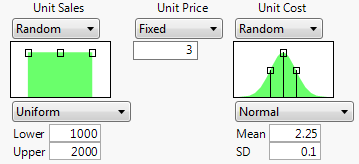7. Click the Simulate button.

Note: Your numbers might differ from those shown in Figure 8.26 due to the random draws in the simulation.

Figure 8.26 Simulator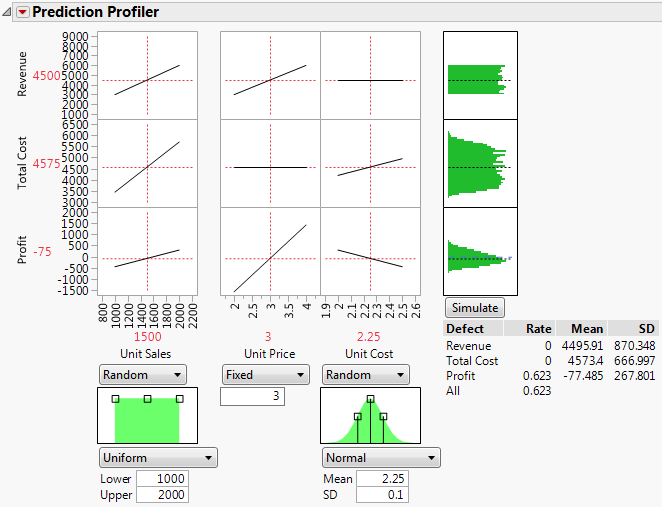It appears that the models are unlikely to be profitable. By putting a lower specification limit of zero on Profit, the defect report tells you that the probability of being unprofitable is 62%.

8. Change the fixed value of Unit Price to 3.25.

9. Click the Simulate button.

Figure 8.27 Results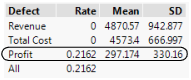Now the probability of being unprofitable is down to about 21%.

If unit price cannot be raised anymore, you should now investigate lowering your cost or increasing sales, if you want to further decrease the probability of being unprofitable.